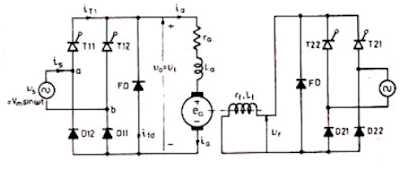## Search This Blog

### Single phase semicoverter drive

• To show in the figure, a separately excited dc motor, fed through two single-phase semi-converter, one for the armature circuit and the other for the field circuit, is shown in the figure.
• Both converter 1, as well as converter 2, are connected to the same single phase source.
• This converter also offers one quadrant drive and it can be used up to about 15 kW dc drives.
• The waveform for current and voltage are sketched in the figure on the assumption of ripple-free armature current.
• Load voltage waveform for V0  = Vt is the same as shown in the figure.
V= V= Vm/∏ * ( 1+  Cos ɖ )

for field circuit, V= V/ ∏ * ( 1+  Cos ɖSingle phase semi converter drive
• To show in the figure, a separately excited dc motor, fed through two single-phase semi-converter, one for the armature circuit and the other for the field circuit, is shown in the figure.
• Both converter 1, as well as converter 2, are connected to the same single phase source.
• This converter also offers one quadrant drive and it can be used up to about 15 kW dc drives.
• The waveform for current and voltage are sketched in the figure on the assumption of ripple-free armature current.
• Load voltage waveform for V0  = Vt is the same as shown in the figure.
V= V= Vm/∏ * ( 1+  Cos ɖ )

for field circuit, V= V/ ∏ * ( 1+  Cos ɖSingle phase semi converter drive